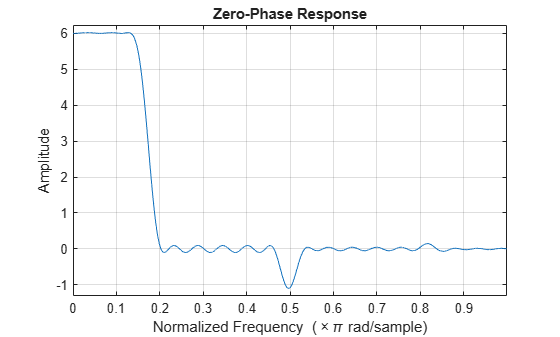# fireqint

Equiripple FIR interpolators

## Syntax

``b = fireqint(n,l,alpha)``
``b = fireqint(n,l,alpha,w)``
``b = fireqint('minorder', l,alpha,r)``
``b = fireqint({'minorder',initord},l,alpha,r)``

## Description

````b = fireqint(n,l,alpha)` designs an FIR equiripple filter useful for interpolating input signals.```
````b = fireqint(n,l,alpha,w)` allows you to specify a vector of weights in `w`.```
````b = fireqint('minorder', l,alpha,r)` allows you to design a minimum-order filter that meets the design specifications. When you use the input argument `'minorder'`, you must provide the maximum ripple vector `r`.```
````b = fireqint({'minorder',initord},l,alpha,r)` allows you to provide an initial estimate of the filter order in the input argument `initord`. Again, you must provide `r`, the vector of maximum deviation or ripples from the ideal filter magnitude response.```

## Examples

collapse all

Design a minimum order interpolation filter with interpolation factor set to `6`, and inverse measure of transition bandwidth set to `0.8`. A vector of ripples must be supplied with the input argument, `minorder`.

`b = fireqint('minorder',6,.8,[0.01 .1 .05 .02]);`

Create a polyphase interpolation filter.

`hm = dsp.FIRInterpolator(6,'Numerator',b);`

Plot the zero-phase response of the interpolator.

`zerophase(hm)`## Input Arguments

collapse all

Order of the equiripple filter, specified as a positive integer. Filter order must be three or more.

Data Types: `double`

Interpolation factor, specified as a positive integer.

Data Types: `double`

Inverse measure of the transition bandwidth, specified as a positive scalar in the range `(0,1)`.

The `alpha` argument is inversely proportional to the transition bandwidth of the filter. It also affects the bandwidth of the don't-care regions in the stopband. Specifying `alpha` allows you to control how much of the Nyquist interval your input signal occupies. This can be beneficial for signals to be interpolated because it allows you to increase the transition bandwidth without affecting the interpolation, resulting in better stopband attenuation for a given `l`. If you set `alpha` argument to `1`, then `fireqint` function assumes that your signal occupies the entire Nyquist interval. Setting the `alpha` argument to a value less than one allows for don't-care regions in the stopband. For example, if your input occupies half the Nyquist interval, you could set the `alpha` to `0.5`.

The signal to be interpolated is assumed to have zero (or negligible) power in the frequency band between (`alpha`*π) and π. The input argument, `alpha` must therefore be a positive scalar between 0 and 1. The `fireqint` function treats such bands as don't-care regions for assessing filter design.

Data Types: `double`

Weights applied to passband ripple and stopband attenuation, specified as a row vector of positive scalars. The number of weights required in `w` is given by 1 + `floor`(`l`/2). Using weights enables you to specify different attenuations in different parts of the stopband, as well as providing the ability to adjust the compromise between passband ripple and stopband attenuation.

Data Types: `double`

Maximum deviations or ripples from the ideal filter magnitude response, specified as a vector. The number of elements required in `r` is given by 1 + `floor`(`l`/2).

Data Types: `double`

Initial filter order when designing a minimum order filter that meets design specifications, specified as a positive integer.

Data Types: `double`

## Output Arguments

collapse all

Filter coefficients of the equiripple filter, returned as a real-valued row vector.

Data Types: `double`

## Version History

Introduced in R2011a